# JS 中 this 在各个场景下的指向

## 1. this 的奥秘

• 函数调用: `alert('Hello World!')`

• 方法调用: `console.log('Hello World!')`

• 构造函数: `new RegExp('\\d')`

• 隐式调用: `alert.call(undefined, 'Hello World!')`

• 函数调用：执行构成函数主体的代码：例如，`parseInt`函数调用是`parseInt('15')`

• 调用的上下文：指 `this` 在函数体内的值。 例如，`map.set('key', 'value')`的调用上下文是 `map`

• 函数的作用域：是在函数体中可访问的变量、对象和函数的集合。

## 2.函数调用

``````function hello(name) {
return 'Hello ' + name + '!';
}
// 函数调用
const message = hello('World');
console.log(message); // => 'Hello World!'

`hello('World')`是函数调用: `hello`表达式等价于一个函数，跟在它后面的是一对括号以及`'World'`参数。

``````const message = (function(name) {
return 'Hello ' + name + '!';
})('World');
console.log(message) // => 'Hello World!'

IIFE也是一个函数调用:第一对圆括号`(function(name) {...})`是一个表达式，它的计算结果是一个函数对象，后面跟着一对圆括号，圆括号的参数是`“World”`

#### 2.1. 在函数调用中的this

this 在函数调用中是一个全局对象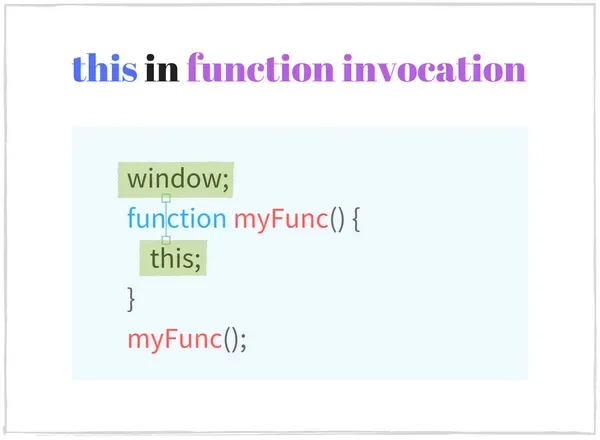``````function sum(a, b) {
console.log(this === window); // => true
this.myNumber = 20; // 将'myNumber'属性添加到全局对象
return a + b;
}
// sum() is invoked as a function
// sum() 中的 `this` 是一个全局对象（window）
sum(15, 16);     // => 31
window.myNumber; // => 20

`this`在任何函数作用域(最顶层作用域:全局执行上下文)之外使用，`this` 表示 `window` 对象

``````console.log(this === window); // => true
this.myString = 'Hello World!';
console.log(window.myString); // => 'Hello World!'

<!-- In an html file -->
<script type="text/javascript">
console.log(this === window); // => true
</script>

#### 2.2 严格模式下的函数调用 this 又是什么样的

`this` 在严格模式下的函数调用中为 undefined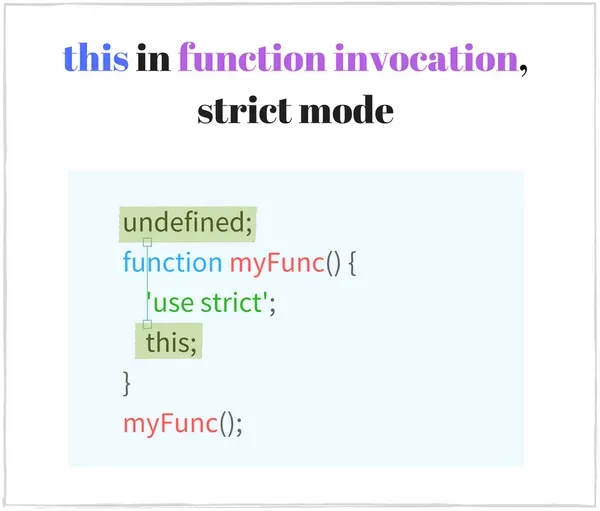``````function multiply(a, b) {
'use strict'; // 启用严格模式
console.log(this === undefined); // => true
return a * b;
}
multiply(2, 5); // => 10

`multiply(2,5)`作为函数调用时，`this``undefined`

``````function execute() {
'use strict'; // 开启严格模式
function concat(str1, str2) {
// 严格模式仍然有效
console.log(this === undefined); // => true
return str1 + str2;
}
// concat() 在严格模式下作为函数调用
// this in concat() is undefined
concat('Hello', ' World!'); // => "Hello World!"
}
execute();

`'use strict'`被插入到执行体的顶部，在其作用域内启用严格模式。 因为函数`concat`是在执行的作用域中声明的，所以它继承了严格模式。

``````function nonStrictSum(a, b) {
// 非严格模式
console.log(this === window); // => true
return a + b;
}
function strictSum(a, b) {
'use strict';
// 启用严格模式
console.log(this === undefined); // => true
return a + b;
}

nonStrictSum(5, 6); // => 11
strictSum(8, 12); // => 20

#### 2.3 陷阱:`this` 在内部函数中的时候

``````const numbers = {
numberA: 5,
numberB: 10,
sum: function() {
console.log(this === numbers); // => true
function calculate() {
console.log(this === numbers); // => false
return this.numberA + this.numberB;
}
return calculate();
}
};
numbers.sum(); // => NaN

`sum()`是对象上的方法调用，所以`sum`中的上下文是`numbers`对象。`calculate`函数是在`sum`中定义的，你可能希望在`calculate()``this`也表示`number`对象。

`calculate()`是一个函数调用(不是方法调用)，它将`this`作为全局对象`window`(非严格模下)。即使外部函数`sum`将上下文作为`number`对象，它在`calculate`里面没有影响。

`sum()`的调用结果是`NaN`，不是预期的结果`5 + 10 = 15`，这都是因为没有正确调用`calculate`

``````const numbers = {
numberA: 5,
numberB: 10,
sum: function() {
console.log(this === numbers); // => true
function calculate() {
console.log(this === numbers); // => true
return this.numberA + this.numberB;
}
// 使用 .call() 方法修改上下文
return calculate.call(this);
}
};
numbers.sum(); // => 15

`call(this)`像往常一样执行`calculate`函数，但 `call` 会把上下文修改为指定为第一个参数的值。

``````const numbers = {
numberA: 5,
numberB: 10,
sum: function() {
console.log(this === numbers); // => true
const calculate = () => {
console.log(this === numbers); // => true
return this.numberA + this.numberB;
}
return calculate();
}
};
numbers.sum(); // => 15

## 3.方法调用

``````const myObject = {
// helloFunction 是一个方法
helloFunction: function() {
return 'Hello World!';
}
};
const message = myObject.helloFunction();

`helloFunction``myObject`的一个方法，要调用该方法，可以这样子调用 :`myObject.helloFunction`

``````['Hello', 'World'].join(', '); // 方法调用
({ ten: function() { return 10; } }).ten(); // 方法调用
const obj = {};
obj.myFunction = function() {
return new Date().toString();
};
obj.myFunction(); // 方法调用

const otherFunction = obj.myFunction;
otherFunction();     // 函数调用
parseFloat('16.60'); // 函数调用
isNaN(0);            // 函数调用

#### 3.1 方法调用中 this 是肿么样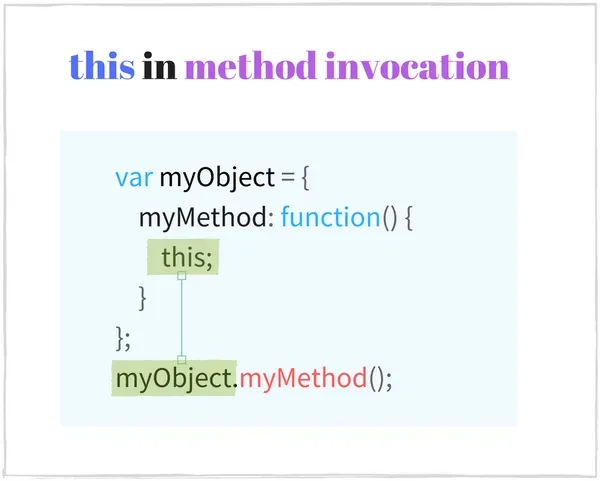``````const calc = {
num: 0,
increment: function() {
console.log(this === calc); // => true
this.num += 1;
return this.num;
}
};
// method invocation. this is calc
calc.increment(); // => 1
calc.increment(); // => 2

``````const myDog = Object.create({
sayName: function() {
console.log(this === myDog); // => true
return this.name;
}
});
myDog.name = 'Milo';
// 方法调用 this 指向 myDog
myDog.sayName(); // => 'Milo'

`Object.create()`创建一个新对象`myDog`，并根据第一个参数设置其原型。`myDog`对象继承`sayName`方法。

``````class Planet {
constructor(name) {
this.name = name;
}
getName() {
console.log(this === earth); // => true
return this.name;
}
}
var earth = new Planet('Earth');
// method invocation. the context is earth
earth.getName(); // => 'Earth'

#### 3.2 陷阱:将方法与其对象分离

``````function Animal(type, legs) {
this.type = type;
this.legs = legs;
this.logInfo = function() {
console.log(this === myCat); // => false
console.log('The ' + this.type + ' has ' + this.legs + ' legs');
}
}
const myCat = new Animal('Cat', 4);
// The undefined has undefined legs
setTimeout(myCat.logInfo, 1000);

``````setTimout(myCat.logInfo);
// 等价于
const extractedLogInfo = myCat.logInfo;
setTimout(extractedLogInfo);

``````function Animal(type, legs) {
this.type = type;
this.legs = legs;
this.logInfo = function() {
console.log(this === myCat); // => true
console.log('The ' + this.type + ' has ' + this.legs + ' legs');
};
}
const myCat = new Animal('Cat', 4);
// logs "The Cat has 4 legs"
setTimeout(myCat.logInfo.bind(myCat), 1000);

`myCat.logInfo.bind(myCat)`返回一个新函数，它的执行方式与`logInfo`完全相同，但是此时的 `this` 指向 `myCat`，即使在函数调用中也是如此。

``````function Animal(type, legs) {
this.type = type;
this.legs = legs;
this.logInfo = () => {
console.log(this === myCat); // => true
console.log('The ' + this.type + ' has ' + this.legs + ' legs');
};
}
const myCat = new Animal('Cat', 4);
// logs "The Cat has 4 legs"
setTimeout(myCat.logInfo, 1000);

## 4. 构造函数调用

`new`关键词紧接着函数对象,(,一组逗号分隔的参数以及)时被调用，执行的是构造函数调用如`new RegExp('\\d')`

``````function Country(name, traveled) {
this.name = name ? name : 'United Kingdom';
this.traveled = Boolean(traveled);
}
Country.prototype.travel = function() {
this.traveled = true;
};
// 构造函数调用
const france = new Country('France', false);
// 构造函数调用
const unitedKingdom = new Country;

france.travel(); // Travel to France

`new Country('France', false)``Country`函数的构造函数调用。它的执行结果是一个`name`属性为`'France'`的新的对象。 如果这个构造函数调用时不需要参数，那么括号可以省略：`new Country`

``````class City {
constructor(name, traveled) {
this.name = name;
this.traveled = false;
}
travel() {
this.traveled = true;
}
}
// Constructor invocation
const paris = new City('Paris', false);
paris.travel();

`new City('Paris')`是构造函数调用。这个对象的初始化由这个类中一个特殊的方法`constructor`来处理。其中，`this`指向新创建的对象。

## 4.1. 构造函数中的 this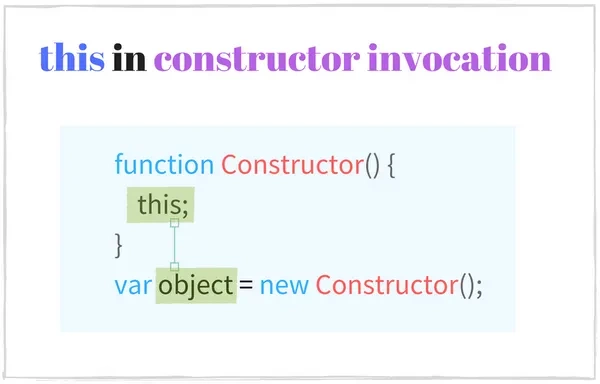``````function Foo () {
console.log(this instanceof Foo); // => true
this.property = 'Default Value';
}
// Constructor invocation
const fooInstance = new Foo();
fooInstance.property; // => 'Default Value'

`new Foo()` 正在进行构造函数调用，其中上下文是`fooInstance`。 在`Foo`内部初始化对象：`this.property`被赋值为默认值。

``````class Bar {
constructor() {
console.log(this instanceof Bar); // => true
this.property = 'Default Value';
}
}
// Constructor invocation
const barInstance = new Bar();
barInstance.property; // => 'Default Value'

#### 4.2. 陷阱: 忘了使用 new

``````var reg1 = new RegExp('\\w+');
var reg2 = RegExp('\\w+');

reg1 instanceof RegExp;      // => true
reg2 instanceof RegExp;      // => true
reg1.source === reg2.source; // => true

``````function Vehicle(type, wheelsCount) {
this.type = type;
this.wheelsCount = wheelsCount;
return this;
}
// 忘记使用 new
const car = Vehicle('Car', 4);
car.type;       // => 'Car'
car.wheelsCount // => 4
car === window  // => true

`Vehicle`是一个在上下文对象上设置`type``wheelsCount`属性的函数。

``````function Vehicle(type, wheelsCount) {
if (!(this instanceof Vehicle)) {
throw Error('Error: Incorrect invocation');
}
this.type = type;
this.wheelsCount = wheelsCount;
return this;
}
// Constructor invocation
const car = new Vehicle('Car', 4);
car.type               // => 'Car'
car.wheelsCount        // => 4
car instanceof Vehicle // => true

// Function invocation. Throws an error.
const brokenCar = Vehicle('Broken Car', 3);

`new Vehicle('Car'，4)` 运行正常：创建并初始化一个新对象，因为构造函数调用中时使用了`new`关键字。

## 5. 隐式调用

JS中的函数是第一类对象，这意味着函数就是对象，对象的类型为`Function`。从函数对象的方法列表中，`.call()``.apply()`用于调用具有可配置上下文的函数。

• 方法 `.call(thisArg[, arg1[, arg2[, ...]]])`将接受的第一个参数`thisArg`作为调用时的上下文，`arg1, arg2, ...`这些则作为参数传入被调用的函数。

• 方法`.apply(thisArg, [args])`将接受的第一个参数`thisArg`作为调用时的上下文，并且接受另一个类似数组的对象`[arg1, arg2, ...] `作为被调用函数的参数传入。

``````function increment(number) {
return ++number;
}
increment.call(undefined, 10);    // => 11
increment.apply(undefined, ); // => 11

`increment.call()``increment.apply()`都用参数`10`调用了这个自增函数。

#### 5.1. 隐式调用中的this

``````var rabbit = { name: 'White Rabbit' };
function concatName(string) {
console.log(this === rabbit); // => true
return string + this.name;
}
concatName.call(rabbit, 'Hello ');  // => 'Hello White Rabbit'
concatName.apply(rabbit, ['Bye ']); // => 'Bye White Rabbit'

``````function Runner(name) {
console.log(this instanceof Rabbit); // => true
this.name = name;
}
function Rabbit(name, countLegs) {
console.log(this instanceof Rabbit); // => true
Runner.call(this, name);
this.countLegs = countLegs;
}
const myRabbit = new Rabbit('White Rabbit', 4);
myRabbit; // { name: 'White Rabbit', countLegs: 4 }

`Rabbit`中的`Runner.call(this, name)`隐式调用了父类的函数来初始化这个对象。

## 6. 绑定函数

``````function multiply(number) {
'use strict';
return this * number;
}
const double = multiply.bind(2);

double(3);  // => 6
double(10); // => 20

`bind(2)`返回一个新的函数对象`double``double` 绑定了数字`2``multiply``double`具有相同的代码和作用域。

`.apply()``.call()` 方法相反，它不会立即调用该函数，`.bind()`方法只返回一个新函数，在之后被调用，只是this`已经`被提前设置好了。

#### 6.1. 绑定函数中的this

`.bind()`的作用是创建一个新函数，调用该函数时，将上下文作为传递给`.bind()`的第一个参数。它是一种强大的技术，使咱们可以创建一个定义了`this`值的函数。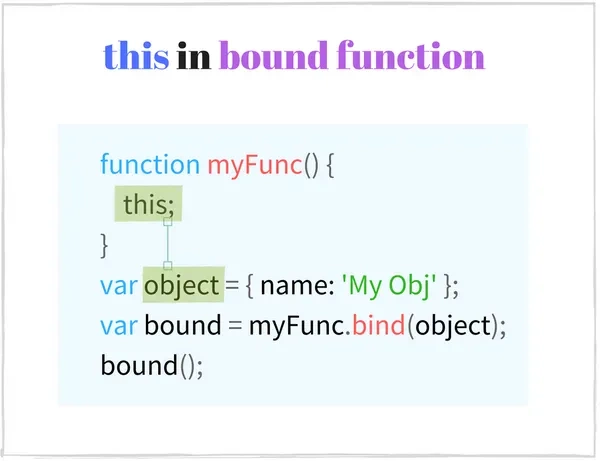``````const numbers = {
array: [3, 5, 10],
getNumbers: function() {
return this.array;
}
};
const boundGetNumbers = numbers.getNumbers.bind(numbers);
boundGetNumbers(); // => [3, 5, 10]
// Extract method from object
const simpleGetNumbers = numbers.getNumbers;
simpleGetNumbers(); // => undefined (严格模式下报错)

`numbers.getNumbers.bind(numbers)`返回绑定`numbers`对象`boundGetNumbers`函数。`boundGetNumbers()`调用时的`this``number`对象，并能够返回正确的数组对象。

#### 6.2 紧密的上下文绑定

`.bind()`创建一个永久的上下文链接，并始终保持它。 一个绑定函数不能通过`.call()`或者`.apply()`来改变它的上下文，甚至是再次绑定也不会有什么作用。

``````function getThis() {
'use strict';
return this;
}
const one = getThis.bind(1);
// 绑定函数调用
one(); // => 1
// 使用带有.apply()和.call()的绑定函数
one.call(2);  // => 1
one.apply(2); // => 1
// 再次绑定
one.bind(2)(); // => 1
// 以构造函数的形式调用绑定函数
new one(); // => Object

## 7. 箭头函数

``````const hello = (name) => {
return 'Hello ' + name;
};
hello('World'); // => 'Hello World'
// Keep only even numbers
[1, 2, 5, 6].filter(item => item % 2 === 0); // => [2, 6]

``````const sumArguments = (...args) => {
console.log(typeof arguments); // => 'undefined'
return args.reduce((result, item) => result + item);
};
sumArguments.name      // => ''
sumArguments(5, 5, 6); // => 16

#### 7.1. 箭头函数中的this

this 定义箭头函数的封闭上下文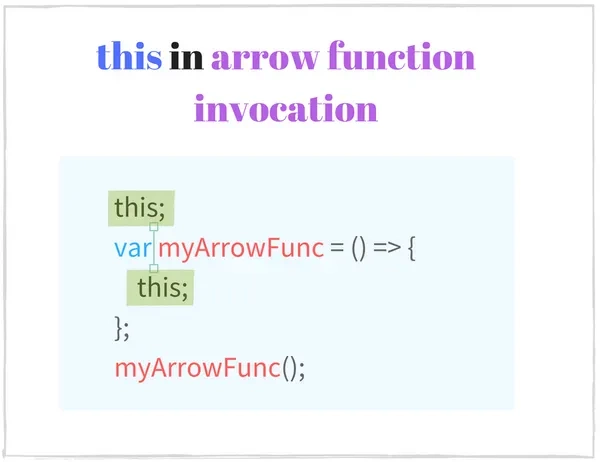``````class Point {
constructor(x, y) {
this.x = x;
this.y = y;
}
log() {
console.log(this === myPoint); // => true
setTimeout(()=> {
console.log(this === myPoint);      // => true
console.log(this.x + ':' + this.y); // => '95:165'
}, 1000);
}
}
const myPoint = new Point(95, 165);
myPoint.log();

`setTimeout`使用与`log()`方法相同的上下文(`myPoint`对象)调用箭头函数。正如所见，箭头函数从定义它的函数继承上下文。

``````onst getContext = () => {
console.log(this === window); // => true
return this;
};
console.log(getContext() === window); // => true

``````const numbers = [1, 2];
(function() {
const get = () => {
console.log(this === numbers); // => true
return this;
};
console.log(this === numbers); // => true
get(); // => [1, 2]
// Use arrow function with .apply() and .call()
get.call();  // => [1, 2]
get.apply(); // => [1, 2]
// Bind
get.bind()(); // => [1, 2]
}).call(numbers);

#### 7.2. 陷阱: 用箭头函数定义方法

``````function Period (hours, minutes) {
this.hours = hours;
this.minutes = minutes;
}
Period.prototype.format = () => {
console.log(this === window); // => true
return this.hours + ' hours and ' + this.minutes + ' minutes';
};
const walkPeriod = new Period(2, 30);
walkPeriod.format(); // => 'undefined hours and undefined minutes'

``````function Period (hours, minutes) {
this.hours = hours;
this.minutes = minutes;
}
Period.prototype.format = function() {
console.log(this === walkPeriod); // => true
return this.hours + ' hours and ' + this.minutes + ' minutes';
};
const walkPeriod = new Period(2, 30);
walkPeriod.format(); // => '2 hours and 30 minutes'

`walkPeriod.format()`是一个对象上的方法调用，它的上下文是`walkPeriod`对象。`this.hours`等于`2``this.minutes`等于`30`，所以这个方法返回了正确的结果：`'2 hours and 30 minutes'`

this 是从哪里来的？